Courses

# Short Answer Type Questions- Constructions Class 10 Notes | EduRev

## Class 10 : Short Answer Type Questions- Constructions Class 10 Notes | EduRev

The document Short Answer Type Questions- Constructions Class 10 Notes | EduRev is a part of the Class 10 Course Class 10 Mathematics by VP Classes.
All you need of Class 10 at this link: Class 10

Q1. Draw a circle of diameter 6.4 cm. Then draw two tangents to the circle from a point P at a distance 6.4 cm from the centre of the circle.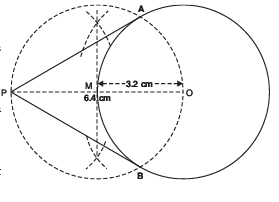Sol. Steps of construction:

I. Draw a circle with centre O and radius = 6.4/2 cm or 3.2 cm.
II. Mark a point P outside the circle such that OP = 6.4 cm.
III. Join OP.
IV. Bisect OP such that its mid point is at M.
V. With centre M and radius OM, draw a circle intersecting the given circle at A and B.
VI. Join PA and PB. Thus, PA and PB are the two tangents to the given circle.

Q2. Draw a circle of radius 3.4 cm. Draw two tangents to it inclined at an angle of 60° to each other: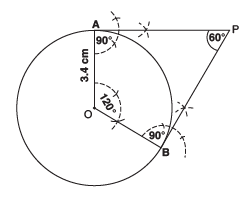Sol. Steps of construction:
I. Draw a circle with centre O and radius as 3.4 cm.
II. Draw two radii OA and OB such that ∠AOB = 120°.
III. Draw perpendiculars at A and B such that these perpendiculars meet at P.
Obviously, ∠APB = 60°. [Using Angle sum property of a quadrilateral]
IV. Thus, PA and PB are the required tangents to the given circle.

Q3. Draw Δ ABC in which AB = 3.8 cm, ∠ B = 60° and median AD = 3.6 cm. Draw another triangle AB’C’ similar to the first such that AB = (4/3)AB.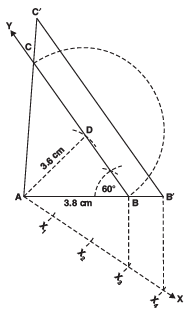Sol. Steps of construction:

I. Draw  AB = 3.8 cm.
II. Construct ∠ABY = 60°.
III. With centre A and radius as 3.6 cm mark a ray to intersect BY at D.
IV. With centre D and radius BD, mark an arc to intersect BY at C.
V. Join CA. Thus, ABC is a triangle.
VI. Draw a ray AX, such that ∠BAX is an acute angle.
VII. Mark 4 points X1, X2, X3 and X4 such that AX1 = X1X2 = X2 X3 = X3 X4.
VIII. Join X3B.
IX. Through X4 draw X4B’ y X3B X. Through B’ draw B’ C’ ║ BC where C′ lies on AC (produced).
Thus, ΔC’AB is the required triangle.

Q4. Draw an equilateral triangle of height 3.6 cm. Draw another triangle similar to it such that its side is 2/3 of the side of the first.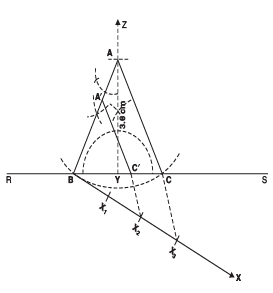Sol. Steps at construction:
I. Draw a line segment RS.
II. Mark a point Y on it.
III. Through Y, draw YZ ⊥ RS
IV. Mark a point A on YZ such that YA = 3.6 cm
V. At A draw ∠YAB = 30° such that the point B is on RS.
VI. With centre A and radius = AB, mark a point C on RS.
VII. Join AC.
VIII. Draw a ray BX such that ∠CBX is an acute angle.
IX. Mark three points X1, X2, X3 such that AX1 = X1X2 = X2 X3.
X. Join X3 and C.
XI. Through X2 draw X2C′ ║ X3C.
XII. Through C′ draw C′ A′ ║ CA.
Thus, ∆A′ BC′ is the required triangle.

Q5. Draw an isosceles ∆ ABC, in which AB = AC = 5.6 cm and ∠ ABC = 60°. Draw another ∆ AB′ C′ similar to ∆ABC such that AB′ = (2/3) AB.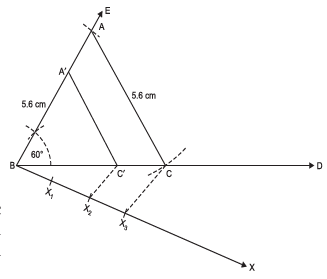Sol. Steps of Construction:
I. Draw a ray BD.
II. Through B, draw another ray BE such that ∠DBE = 60°.
III. Cut off BA = 5.6 cm.
IV. With A as centre and radius 6 cm, mark an arc intersecting BD at C.
V. Join A and C to get ΔABC.
VI. Draw a ray BX such that ∠CBX is an acute angle.
VII. Mark three point X1, X2 and X3 such that BX1 = X1X2 = X2X3.
VIII. Join X3 and C.
IX. Through X2 draw X2C′ ║ X3C
X. Through C′ , draw C′ A′ ║ CA
Thus, Δ A′ BC′ is the required triangle.

Q6. Construct an isosceles triangle whose base is 9 cm and altitude is 5 cm. Then construct another triangle whose sides are 3/4 of the corresponding sides of the first isosceles triangle.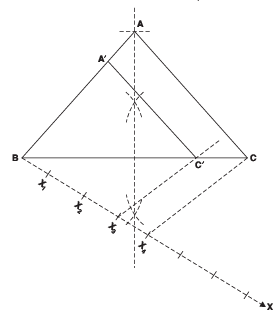Sol. Steps of construction:
I. Construct a ΔABC such that AB = AC, BC = 9 cm and altitude AD = 5 cm.
II. Through B, draw a ray BX such that ∠CBX is an acute angle.
III. Mark 4 equal points X1, X2, X3 and X4 on BX. such that BX1 = X1 X2 = X2 X3 = X3 X4
IV. Join X4 and C.
V. Through X3, draw X3C′ ║ X4C, intersecting BC in C′.
VI. Through C′ , draw C′ A′ ║ CA, intersecting AB in A′.
Thus, ΔA′BC′ is the required triangle.

Q7. Draw a line segment AB of length 7 cm. Taking A as centre draw a circle of radius 3 cm and taking B as centre, draw another circle of radius 2.5 cm. Construct tangents to each circle from the centre of the other circle.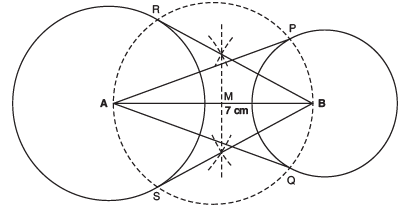Sol. Steps of construction:
I. Draw a line segment AB = 7 cm
II. With centre A and radius 3 cm, draw a circle.
III. With centre B and radius 2.5 cm, draw another circle.
IV. Bisect AB and let M be the mid point of AB.
V. With centre M and radius AM, draw a circle intersecting the two circles in P,Q and R,S.
VI. Join AP, AQ, BR and BS.
Thus, AP, AQ, BR and BS are required tangents.

Q8. Construct a Δ ABC in which BC = 6.5 cm, AB = 4.5 cm and ∠ABC = 60°. Construct a triangle similar to this triangle whose sides are 3/4 of the corresponding sides of the ΔABC.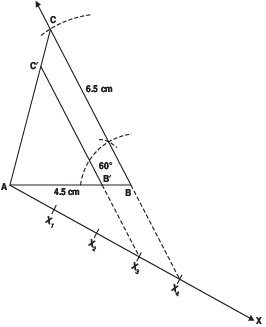Sol. Steps of construction:

I. Construct the ΔABC such that AB = 4.5 cm, ∠B = 60° and BC = 6.5 cm.
II. Construct an acute angle ∠BAX.
III. Mark 4 points X1, X2, X3 and X4 on AX such that AX1 = X1X2 = X2X3 =X3X4.
IV. Join X4 and B.
V. Draw X3 B′ ║ BC, meeting AC at C′ .
Thus, ΔC′AB′ is the required Δ.

Q9. Draw a right triangle in which sides (other than hypotenuse) are of lenghts 8 cm and 6 cm. Then construct another triangle whose sides are 3/4 times the corresponding sides of the first triangle.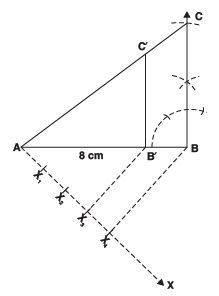Sol. Steps of construction:
I. Draw a ΔABC such that AB = 8 cm, ∠B = 90° and BC = 6 cm.
II. Construct an acute angle ∠BAX.
III. Mark 4 points X1, X2, X3 and X4 on AX such that AX1 = X1X2, = X2X3 = X3X4.
IV. Join X4 and B.
V. Draw X3B′ ║ X4B.
VI. Draw B′ C′ ║ BC.
Thus, ΔAB′C′ is the required rt Δ.

Q10. Construct a ΔABC in which BC = 5 cm, CA = 6 cm and AB = 7 cm. Construct a ΔA′ BC′ similar to ΔABC, each of whose sides are 7/5 times the corresponding sides of ΔABC.

Sol. Steps of construction:
I. Construct ΔABC such that: BC = 5 cm, CA = 6 cm and AB = 7 cm.
II. Draw a ray BX such that ∠CBX is an acute angle.
III. Mark 7 points X1, X2, .............. X7 such that: BX1 = X1X2, = X2X3 = X3X4 = X4X5 = X5X6 = X6X7
IV. Join X7 and C.
V. Draw a line through X5 parallel to X7C to meet BC extended at C′ .
VI. Through C′ , draw a line parallel to CA to meet BA extended at A′ .

Thus, ΔA′BC′ is the required triangle.

Q11. Construct a triangle with sides 4 cm, 5 cm and 7 cm. Then construct a triangle similar to it whose sides are 2/3 of the corresponding sides of the given triangle.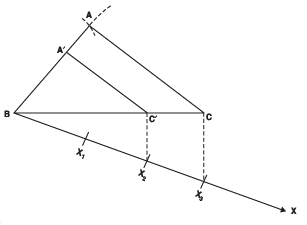Sol. Steps of construction:
I. Construct the ΔABC such that BC = 7 cm, CA = 5 cm and BA = 4 cm.
II. Draw a ray BX such that ∠CBX is an acute angle.
III. Mark three points X1, X2 and X3 on BX such that: BX1 = X1 X2 = X2 X3
IV. Join X3 and C.
V. Draw X2C′ ║ X3C.
VI. Draw C′ A′ ║ CA

Thus, ΔA′ BC′ is the required triangle.

Q12. Construct a ΔABC in which AB = 6.5 cm, ∠ B = 60° and BC = 5.5 cm. Also construct a triangle AB′C′ similar to ΔABC whose each side is 3/2 times the corresponding side of the ΔABC.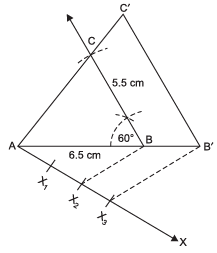Sol. Steps of construction:
I. Construct a ΔABC such that AB = 6.5 cm, ∠B = 60° and BC = 5.5 cm.
II. Draw a ray AX making an acute angle ∠BAX.
III. Mark three points X1, X2, X3 on the ray AX such that AX1 = X1 X2 = X2 X3
IV. Join X2 and B.
V. Draw X3B′ ║ X2B such that B′ is a point on extended AB.
VI. Join B′ C′ y BC such that C′ is a point on AC (extended).
Thus, ΔC′ AB′ is the required triangle.

Q13. Draw a ΔABC with side BC = 6 cm, AB = 5 cm and ∠ ABC = 60°. Construct ΔAB′ C′ similar to ΔABC such that sides of ΔAB′C′ are 3/4 of the corresponding sides of ΔABC.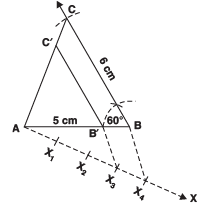Sol. Steps of construction:

I. Construct the given ΔABC.
II. Draw a ray AX such that ∠BAC is an acute angle.
III. Mark 4 points X1, X2, X3 and X4 on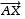such that AX1 = X1 X2 = X2 X3 = X3 X4.
IV. Join X4 B.
V. Draw X3B′ ║ X4B
VI. Through B′ draw B′ C′ ║ BC.
Thus, ΔB′ AC′ is the required triangle.

Q14. Draw a circle of radius 3 cm. From a point P, 6 cm away from its centre, construct a pair of tangents to the circle. Measure the lengths of the tangents.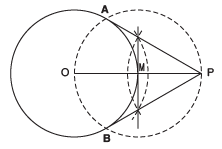Sol. Steps of construction:

I. Draw the given circle such that its centre is at O and radius = 3 cm.
II. Mark a point P such that OP = 6 cm.
III. Bisect OP. Let M be the mid point of OP.
IV. Taking M as centre and OM as radius draw a circle intersecting the given circle at A and B.
V. Join PA and PB.
Thus, PA and PB are the required tangents to the given circle.

Q15. Construct a triangle whose perimeter is 13.5 cm and the ratio of the three sides is 2 : 3 : 4.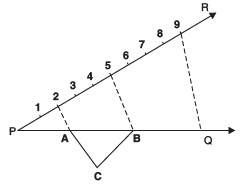Sol. Steps of construction:
I. Draw a line PQ = 13.5 cm
II. At P, draw a ray PR making a convenient acute angle –QPR with PQ.
III. On PR mark (2 + 3 + 4), 9 points at equal distances.
IV. Join Q and the mark 9.
V. Through the points 2 and 5 draw lines 2-A and 5-B parallel to 9-Q. Let these lines meet PQ at A and B respectively.
VI. With A as centre and radius = AP, draw an arc.
VII. With B as centre and radius = BQ, draw another arc which intersects the arc of step
VI at C.
VIII. Join CA and CB.
ABC is the required triangle.

Offer running on EduRev: Apply code STAYHOME200 to get INR 200 off on our premium plan EduRev Infinity!

132 docs

,

,

,

,

,

,

,

,

,

,

,

,

,

,

,

,

,

,

,

,

,

;